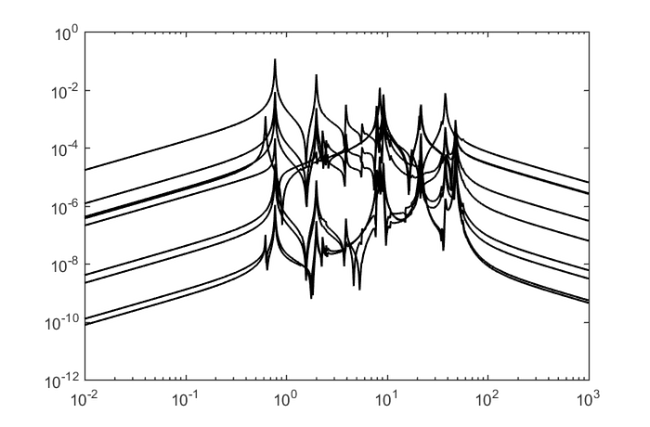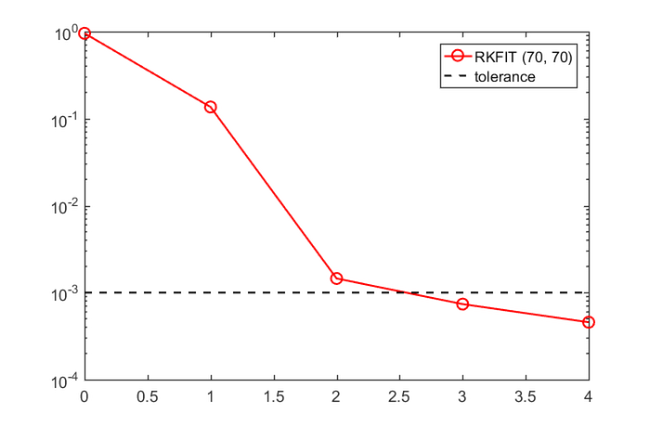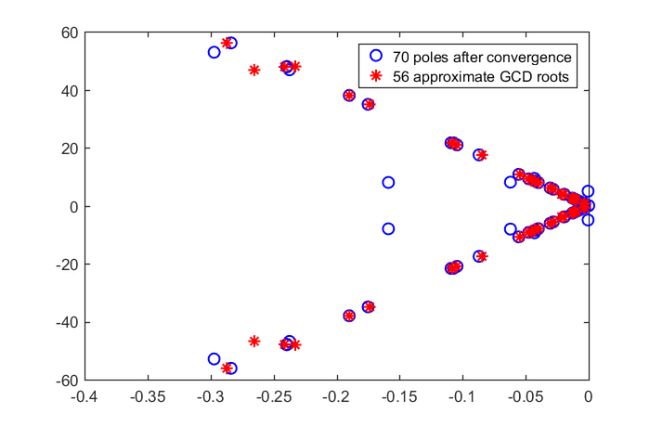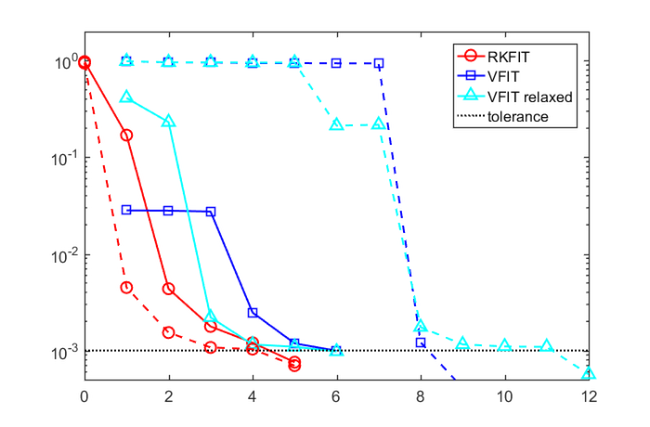# International Space Station model

## Introduction

In this example we show how to use RKFIT [2, 3] from the RK Toolbox  to compute a lower-order model for the multiple-input/multiple-output dynamical system ISS 1R taken from . We demonstrate the automated degree reduction capabilities of RKFIT, as well as its faster convergence compared to VFIT [5, 6, 7]. This example is explained in detail in [3, Section 6.1].

We now load the data and construct the matrices in real form as explained in [3, Section 3.5]. There are 3 input and 3 output channels, giving a total of $\ell = 9$ functions to fit. The frequencies we evaluate at are given in the vector w.

if exist('iss.mat') ~= 2
disp(['http://slicot.org/20-site/126-benchmark-examples' ...
'-for-model-reduction'])
return
end

ell = 3*3;
N   = 2*length(w);
F   = zeros(N/2, ell);

for j = 1:N/2
% We now evaluate the responses at 1i*w(j) from the state
% space representation. The responses at -1i*w(j) is the
% conjugated one.
resp = full(C*((A-1i*w(j)*speye(length(A)))\B));
F(j, :) = resp(:).';
end

Amat = util_build_real_matrix(1i*w);
for j = 1:ell
Fmat{j} = util_build_real_matrix(F(:, j));
end


The following plot shows the magnitudes of the $\ell$ transfer functions, over the frequency range w.

figure
for j = 1:ell
loglog(w, abs(F(:, j)), 'k-'), hold on
end## RKFIT and degree reduction

We search for approximants of type $(70, 70)$ to reach a relative misfit below $10^{-3}$ in at most $10$ iterations. We use the real version, and impose no spectral weighting.

m   = 70;
tol = 1e-3;
b   = zeros(N, 1);
b(1:2:end) = 1;

init = util_ieee_poles(-2, 3, 1e-2, 70);
[xi, ~, misfit, out] = rkfit(Fmat, Amat, b, init, ...
struct('maxit',     10, ...
'tol',       tol, ...
'real',      1, ...
'k',         0, ...
'safe',      1, ...
'stable',    0, ...
'reduction', 1));


The next plot shows the progress of the misfit during the iterations. For the first iteration (index $0$) we show the misfit corresponding to the initial choice of poles.

figure
iter = length(misfit);
semilogy(0:iter,   [out.misfit_initial misfit], 'ro-'), hold on
semilogy([0 iter], [tol tol], 'k--')
legend('RKFIT (70, 70)' , 'tolerance')After the fourth iteration a reduction of $m=70$ occurs. The misfit listed for iteration $5$ correspond to the misfit with the newly selected $m-\Delta m = 56$ poles, as it is below the tolerance, no further RKFIT iterations are required. The $70$ poles, and the $56$ corresponding to the approximate GCD of the $15$-dimensional nullspace are plotted in the next graph.

figure
plot(real(out.xi_unreduced), imag(out.xi_unreduced), 'bo')
hold on
plot(real(out.xi_reduced),   imag(out.xi_reduced),   'r*')
legend('70 poles after convergence', '56 approximate GCD roots')
axis([-.4,0,-60,60])To demonstrate the benefit of the pole-selection process after the reduction we add a plot of the convergence of RKFIT with all initial $56$ poles chosen without the acquired information, in a way similar to that of choosing the $70$ poles before.

m = 56;

init = util_ieee_poles(-2, 3, 1e-2, m);
[xi, ~, misfit56, out56] = rkfit(Fmat, Amat, b, init, ...
struct('maxit', 10, ...
'tol',       tol, ...
'real',      1, ...
'k',         0, ...
'safe',      1, ...
'stable',    0, ...
'reduction', 0));

figure
iter = length(misfit56);
semilogy(0:iter,  [out56.misfit_initial misfit56],'ro-'), hold on
semilogy([0 iter],[tol tol], 'k--')
legend('RKFIT (56, 56)' , 'tolerance')## Comparison with VFIT

We now compare RKFIT and VFIT. The vectfit3 implementation of VFIT is assumed to be available on MATLAB's search path.

if exist('vectfit3') ~= 2
return
end


We use $m=56$ again, and perform $15$ iterations with VFIT. Both VFIT with relaxed nontriviality constraint , and the original version with asymptotic nontriviality constraint  are tested. For the comparison we use two different sets of starting poles, and run RKFIT and the two versions of VFIT with them. The following plot shows the convergence results for both, with the iterations being stopped when the tolerance is reached.

% Options for VFIT.
opts_a.spy1      = 0; opts_a.spy2      = 0;
opts_a.errplot   = 0; opts_a.phaseplot = 0;
opts_a.skip_pole = 0; opts_a.skip_res  = 0;
opts_a.cmplx_ss  = 0; opts_a.legend    = 0;
opts_a.asymp     = 2;
opts_a.stable    = 0;
opts_a.relax     = 0;

opts_r = opts_a;
opts_r.relax = 1;

% Run tests for VFIT with the same poles as for RKFIT.
xi = init; xi_r = init;
for j = 1:15
[~, xi, ~, fit] = vectfit3(F.', 1i*w.', xi, ...
ones(1, length(w)), opts_a);
[~, xi_r, ~, fit_r] = vectfit3(F.', 1i*w.', xi_r, ...
ones(1, length(w)), opts_r);

vfit_a(j) = norm(fit-F.', 'fro')/norm(F, 'fro');
vfit_r(j) = norm(fit_r-F.', 'fro')/norm(F, 'fro');
end

figure(5)
len_a = min(find(vfit_a<1e-3));
len_r = min(find(vfit_r<1e-3));

semilogy(0:iter,   [out56.misfit_initial misfit56], 'ro-'), hold on
semilogy(1:len_a, vfit_a(1:len_a), 'bs-'), hold on
semilogy(1:len_r, vfit_r(1:len_r), 'c^-'), hold on
semilogy([0 100], [tol tol], 'k:')
legend('RKFIT', 'VFIT', 'VFIT relaxed', 'tolerance')

% Choose a new set of starting poles and repeat all three tests.
rng(0)
init = eig(rand(m)-10*eye(m));
[xi, ~, misfit56, out56] = rkfit(Fmat, Amat, b, init, ...
struct('maxit', 10, ...
'tol',       tol, ...
'real',      1, ...
'k',         0, ...
'safe',      1, ...
'stable',    0, ...
'reduction', 0));
xi = init; xi_r = init;
for j = 1:15
[~, xi, ~, fit] = vectfit3(F.', 1i*w.', xi, ...
ones(1, length(w)), opts_a);
[~, xi_r, ~, fit_r] = vectfit3(F.', 1i*w.', xi_r, ...
ones(1, length(w)), opts_r);

vfit_a(j) = norm(fit-F.', 'fro')/norm(F, 'fro');
vfit_r(j) = norm(fit_r-F.', 'fro')/norm(F, 'fro');
end

iter2  = length(misfit56);
len_a2 = min(find(vfit_a<1e-3));
len_r2 = min(find(vfit_r<1e-3));

semilogy(1:len_a2, vfit_a(1:len_a2), 'bs--'), hold on
semilogy(1:len_r2, vfit_r(1:len_r2), 'c^--'), hold on
semilogy(0:iter2, [out56.misfit_initial misfit56], 'ro--'), hold on
xlim([0 max([len_a, len_r, len_a2, len_r2])]), ylim([5e-4,2])

> In vectfit3 (line 423)
In example_iss (line 162)
In evalmxdom>instrumentAndRun (line 109)
In evalmxdom (line 21)
In publish (line 189)
In x_examplepublish (line 96)
Warning: Rank deficient, rank = 44, tol =  1.119105e-13.
> In vectfit3 (line 364)
In example_iss (line 164)
In evalmxdom>instrumentAndRun (line 109)
In evalmxdom (line 21)
In publish (line 189)
In x_examplepublish (line 96)
Warning: Rank deficient, rank = 45, tol =  1.139089e-13.
> In vectfit3 (line 423)
In example_iss (line 162)
In evalmxdom>instrumentAndRun (line 109)
In evalmxdom (line 21)
In publish (line 189)
In x_examplepublish (line 96)
Warning: Rank deficient, rank = 55, tol =  1.119105e-13.
> In vectfit3 (line 364)
In example_iss (line 164)
In evalmxdom>instrumentAndRun (line 109)
In evalmxdom (line 21)
In publish (line 189)
In x_examplepublish (line 96)
Warning: Rank deficient, rank = 54, tol =  1.139089e-13.
> In vectfit3 (line 423)
In example_iss (line 194)
In evalmxdom>instrumentAndRun (line 109)
In evalmxdom (line 21)
In publish (line 189)
In x_examplepublish (line 96)
Warning: Rank deficient, rank = 14, tol =  1.119105e-13.
> In vectfit3 (line 629)
In example_iss (line 194)
In evalmxdom>instrumentAndRun (line 109)
In evalmxdom (line 21)
In publish (line 189)
In x_examplepublish (line 96)
Warning: Rank deficient, rank = 22, tol =  2.491340e-13.
> In vectfit3 (line 364)
In example_iss (line 196)
In evalmxdom>instrumentAndRun (line 109)
In evalmxdom (line 21)
In publish (line 189)
In x_examplepublish (line 96)
Warning: Rank deficient, rank = 15, tol =  1.139089e-13.
> In vectfit3 (line 629)
In example_iss (line 196)
In evalmxdom>instrumentAndRun (line 109)
In evalmxdom (line 21)
In publish (line 189)
In x_examplepublish (line 96)
Warning: Rank deficient, rank = 22, tol =  2.491340e-13.
> In vectfit3 (line 423)
In example_iss (line 194)
In evalmxdom>instrumentAndRun (line 109)
In evalmxdom (line 21)
In publish (line 189)
In x_examplepublish (line 96)
Warning: Rank deficient, rank = 20, tol =  1.119105e-13.
> In vectfit3 (line 629)
In example_iss (line 194)
In evalmxdom>instrumentAndRun (line 109)
In evalmxdom (line 21)
In publish (line 189)
In x_examplepublish (line 96)
Warning: Rank deficient, rank = 33, tol =  2.491340e-13.
> In vectfit3 (line 364)
In example_iss (line 196)
In evalmxdom>instrumentAndRun (line 109)
In evalmxdom (line 21)
In publish (line 189)
In x_examplepublish (line 96)
Warning: Rank deficient, rank = 21, tol =  1.139089e-13.
> In vectfit3 (line 629)
In example_iss (line 196)
In evalmxdom>instrumentAndRun (line 109)
In evalmxdom (line 21)
In publish (line 189)
In x_examplepublish (line 96)
Warning: Rank deficient, rank = 33, tol =  2.491340e-13.
> In vectfit3 (line 423)
In example_iss (line 194)
In evalmxdom>instrumentAndRun (line 109)
In evalmxdom (line 21)
In publish (line 189)
In x_examplepublish (line 96)
Warning: Rank deficient, rank = 30, tol =  1.119105e-13.
> In vectfit3 (line 629)
In example_iss (line 194)
In evalmxdom>instrumentAndRun (line 109)
In evalmxdom (line 21)
In publish (line 189)
In x_examplepublish (line 96)
Warning: Rank deficient, rank = 40, tol =  2.491340e-13.
> In vectfit3 (line 364)
In example_iss (line 196)
In evalmxdom>instrumentAndRun (line 109)
In evalmxdom (line 21)
In publish (line 189)
In x_examplepublish (line 96)
Warning: Rank deficient, rank = 31, tol =  1.139089e-13.
> In vectfit3 (line 629)
In example_iss (line 196)
In evalmxdom>instrumentAndRun (line 109)
In evalmxdom (line 21)
In publish (line 189)
In x_examplepublish (line 96)
Warning: Rank deficient, rank = 38, tol =  2.491340e-13.
> In vectfit3 (line 423)
In example_iss (line 194)
In evalmxdom>instrumentAndRun (line 109)
In evalmxdom (line 21)
In publish (line 189)
In x_examplepublish (line 96)
Warning: Rank deficient, rank = 36, tol =  1.119105e-13.
> In vectfit3 (line 629)
In example_iss (line 194)
In evalmxdom>instrumentAndRun (line 109)
In evalmxdom (line 21)
In publish (line 189)
In x_examplepublish (line 96)
Warning: Rank deficient, rank = 47, tol =  2.491340e-13.
> In vectfit3 (line 364)
In example_iss (line 196)
In evalmxdom>instrumentAndRun (line 109)
In evalmxdom (line 21)
In publish (line 189)
In x_examplepublish (line 96)
Warning: Rank deficient, rank = 37, tol =  1.139089e-13.
> In vectfit3 (line 629)
In example_iss (line 196)
In evalmxdom>instrumentAndRun (line 109)
In evalmxdom (line 21)
In publish (line 189)
In x_examplepublish (line 96)
Warning: Rank deficient, rank = 47, tol =  2.491340e-13.
> In vectfit3 (line 423)
In example_iss (line 194)
In evalmxdom>instrumentAndRun (line 109)
In evalmxdom (line 21)
In publish (line 189)
In x_examplepublish (line 96)
Warning: Rank deficient, rank = 45, tol =  1.119105e-13.
> In vectfit3 (line 629)
In example_iss (line 194)
In evalmxdom>instrumentAndRun (line 109)
In evalmxdom (line 21)
In publish (line 189)
In x_examplepublish (line 96)
Warning: Rank deficient, rank = 52, tol =  2.491340e-13.
> In vectfit3 (line 364)
In example_iss (line 196)
In evalmxdom>instrumentAndRun (line 109)
In evalmxdom (line 21)
In publish (line 189)
In x_examplepublish (line 96)
Warning: Rank deficient, rank = 45, tol =  1.139089e-13.
> In vectfit3 (line 629)
In example_iss (line 196)
In evalmxdom>instrumentAndRun (line 109)
In evalmxdom (line 21)
In publish (line 189)
In x_examplepublish (line 96)
Warning: Rank deficient, rank = 51, tol =  2.491340e-13.
> In vectfit3 (line 423)
In example_iss (line 194)
In evalmxdom>instrumentAndRun (line 109)
In evalmxdom (line 21)
In publish (line 189)
In x_examplepublish (line 96)
Warning: Rank deficient, rank = 49, tol =  1.119105e-13.
> In vectfit3 (line 364)
In example_iss (line 196)
In evalmxdom>instrumentAndRun (line 109)
In evalmxdom (line 21)
In publish (line 189)
In x_examplepublish (line 96)
Warning: Rank deficient, rank = 49, tol =  1.139089e-13.
> In vectfit3 (line 629)
In example_iss (line 196)
In evalmxdom>instrumentAndRun (line 109)
In evalmxdom (line 21)
In publish (line 189)
In x_examplepublish (line 96)
Warning: Rank deficient, rank = 56, tol =  2.491340e-13.
> In vectfit3 (line 423)
In example_iss (line 194)
In evalmxdom>instrumentAndRun (line 109)
In evalmxdom (line 21)
In publish (line 189)
In x_examplepublish (line 96)
Warning: Rank deficient, rank = 55, tol =  1.119105e-13.
> In vectfit3 (line 364)
In example_iss (line 196)
In evalmxdom>instrumentAndRun (line 109)
In evalmxdom (line 21)
In publish (line 189)
In x_examplepublish (line 96)
Warning: Rank deficient, rank = 55, tol =  1.139089e-13.Note: The above warnings outputted by VFIT are due to ill-conditioned linear algebra problems being solved, a problem that is circumvented with RKFIT by the use of discrete-orthogonal rational basis functions.

## References

 M. Berljafa and S. Güttel. A Rational Krylov Toolbox for MATLAB, MIMS EPrint 2014.56 (http://eprints.ma.man.ac.uk/2390/), Manchester Institute for Mathematical Sciences, The University of Manchester, UK, 2014.

 M. Berljafa and S. Güttel. Generalized rational Krylov decompositions with an application to rational approximation, SIAM J. Matrix Anal. Appl., 36(2):894--916, 2015.

 M. Berljafa and S. Güttel. The RKFIT algorithm for nonlinear rational approximation, SIAM J. Sci. Comput., 39(5):A2049--A2071, 2017.

 Y. Chahlaoui and P. Van Dooren. A collection of benchmark examples for model reduction of linear time invariant dynamical systems, MIMS EPrint 2008.22 (http://eprints.ma.man.ac.uk/1040/), Manchester Institute for Mathematical Sciences, The University of Manchester, UK, 2008.

 D. Deschrijver, M. Mrozowski, T. Dhaene, and D. De Zutter. Macromodeling of multiple systems using a fast implementation of the vector fitting method, IEEE Microw. Compon. Lett., 18(6):383--385, 2008.

 B. Gustavsen. Improving the pole relocating properties of vector fitting, IEEE Trans. Power Del., 21(3):1587--1592, 2006.

 B. Gustavsen and A. Semlyen. Rational approximation of frequency domain responses by vector fitting, IEEE Trans. Power Del., 14(3):1052--1061, 1999.